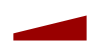Reflexxes Motion Libraries  Manual and Documentation (Type II, Version 1.2.6)
Safety: Three Layers of Error Handling

If one the methods

is called with valid input values, it is guaranteed that the output values of the Reflexxes algorithms lead to a continuous acceleration-limited motion trajectory in any case. This fundamental feature was implemented using a three-layered approach of error-handling, which is described in the following two sections.

## Error-Handling for the Position-based On-Line Trajectory Generation Algorithm

The position-based Reflexxes Type II On-Line Trajectory Generation (OTG) algorithm is the most complex algorithm of the Reflexxes Motion Libraries. Robustness and numerical stability are key features to assure deterministic and safe behaviors of robots and mechanical systems. After more than eight years of research and development, the released algorithm runs robust and is numerically stable.

In order to guarantee continuous acceleration-limited motion trajectories, three layers of error-handling have been implemented in order to obtain a deterministic and safe behavior of all Reflexxes Motion Libraries for any set given input values.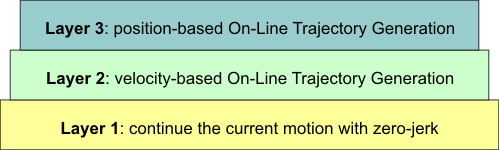Three layers of safety and error-handling for the position-based On-Line Trajectory Generation algorithm.

### Layer 3

This layer contains the position-based Reflexxes Type II On-Line Trajectory Generation algorithm. This algorithm is called through the API method ReflexxesAPI::RMLPosition(), which calls the internal method TypeIIRMLPosition::GetNextStateOfMotion() that contains the actual position-based trajectory generation algorithm. If the algorithm is fed with valid input values, that is, the method RMLPositionInputParameters::CheckForValidity() returns false, we switch from Layer 3 to Layer 2 (even after after more than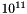cylces, this has not happened yet).

The implementation of Layer 2 can be found in the method TypeIIRMLPosition::FallBackStrategy().

### Layer 2

In the second layer, the velocity-based algorithm of the Reflexxes Type II Motion Library is executed in order to safely transfer the system to a predefined velocity (cf. ReflexxesAPI::RMLVelocity()). The default value for this alternative desired velocity vector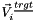is zero, that is, the robot or mechanical system would simply slow down to zero-velocity by using the currently given maximum acceleration vector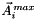(cf. RMLPositionInputParameters::MaxAccelerationVector) until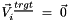is reached. In order to decouple all selected degrees of freedom and to reach this sate safely, a non-synchronized motion will be computed, and the flag RMLFlags::NO_SYNCHRONIZATION will be used for the velocity-based algorithm.

If it is not desired to decrease the velocity to zero but to continue moving the system with a certain application- or task-dependent velocity, an alternative desired target velocity vectorcan be defined. The attribute RMLPositionInputParameters::AlternativeTargetVelocityVector can be used to specify this alternative velocity vector unequal to zero,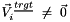. Furthermore, the input flag RMLPositionFlags::KeepCurrentVelocityInCaseOfFallbackStrategy can be used to set the alternative desired target velocity vector to the current velocity vector, that is,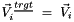.

The mathematical equations of the velocity-based On-Line Trajectory Generation only contain closed-form solutions; numerical instabilities cannot occur if the Input Requirements for Numerical Stability are met. In case, the user specifies elements of the maximum acceleration vectorthat are equal or less than zero (cf. RMLPositionInputParameters::MaxAccelerationVector), the algorithm will even switch from Layer 2 to Layer 1 in order to continue the current motion. This layer is implemented in the method TypeIIRMLVelocity::FallBackStrategy().

### Layer 1

Although even the probability of switching from Layer 3 to Layer 2 can only happen in case of incorrect input values, a third layer is used to handle this case. In this case, the motion of the system is continued with zero-acceleration. That means the output values RMLPositionOutputParameters determined by the following equations: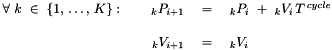Where the variables and symbols represent the following data:

•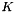is the number of degrees of freedom of the system

•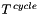is the cycle time given in seconds

•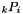is the position of the degree of freedom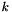at time instant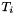•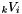is the velocity of the degree of freedomat time instant•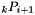is the position of the degree of freedomat time instant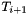•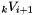is the velocity of the degree of freedomat time instant• The time difference from one control cycle atto the next control cycle atis, that is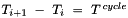.

Details about the implementation of these equations can be found in the method TypeIIRMLVelocity::FallBackStrategy().

### Remark on Phase-Synchronized Trajectories

If a phase-synchronized motion trajectory is required (i.e., the input flag RMLPositionFlags::ONLY_PHASE_SYNCHRONIZATION is set), and if phase-synchronisation is not possible because the input vectors are not collinear, we also switch from Layer 3 to Layer 2, because in this case, priority is given to the fact that phase-synchronization is required (otherwise, the flag RMLPositionFlags::PHASE_SYNCHRONIZATION_IF_POSSIBLE may be used). If we switch to the second layer in this case, the algorithm will check, whether a phase-synchronous solution is existent if the velocity-based On-Line Trajectory Generation is executed. If so, the velocity-based algorithm will compute a phase-synchronized motion. Otherwise, we will switch from Layer 2 to Layer 1. If the current state of motion is homothetic, the trajectory will be continued homothetically (phase-synchronized) by applying zero-acceleration in the current control cycle.

## Error-Handling for the Velocity-based On-Line Trajectory Generation Algorithm

The velocity-based Reflexxes Type II On-Line Trajectory Generation (OTG) algorithm is of much simpler nature than the position-based algorithm and only a two-layered method is used for error-handling. This safety mechanism is directly derived from Layer 2 and Layer 1 of the position-based On-Line Trajectory Generation algorithm.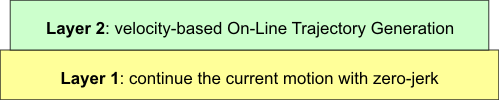Two layers of safety and error-handling for the velocity-based On-Line Trajectory Generation algorithm.

The algorithm is called through the API method ReflexxesAPI::RMLVelocity(), which executes the internal method TypeIIRMLVelocity::GetNextStateOfMotion(). If the input values for this algorithm are valid, that is, the result of RMLVelocityInputParameters::CheckForValidity() is true, the velocity-based algorithm will always find the correct solution. If RMLVelocityInputParameters::CheckForValidity() returns false, because elements of the maximum acceleration vectorthat or equal or less than zero (cf. RMLVelocityInputParameters::MaxAccelerationVector and RMLVelocityInputParameters::MaxJerkVector), the algorithm will switch from Layer 2 to Layer 1 in order to continue the current motion. This layer is implemented in the method TypeIIRMLVelocity::FallBackStrategy(). Please refer to Sec. Layer 1 of the position-based algorithm for a detailed description.

### Remark on Phase-Synchronized Trajectories

If a phase-synchronized motion trajectory is required (i.e., the input flag RMLVelocityFlags::ONLY_PHASE_SYNCHRONIZATION is set), and if phase-synchronisation is not possible because the input vectors are not collinear, we also apply the switching mechanism described above (i.e., the new state of motion is computed by applying a zero-acceleration). If the current state of motion is homothetic, the trajectory will be continued homothetically (phase-synchronized).

User documentation of the Reflexxes Motion Libraries by Reflexxes GmbH (Company Information, Impressum). This document was generated with Doxygen on Mon Jul 7 2014 13:21:09. Copyright 2010–2014.